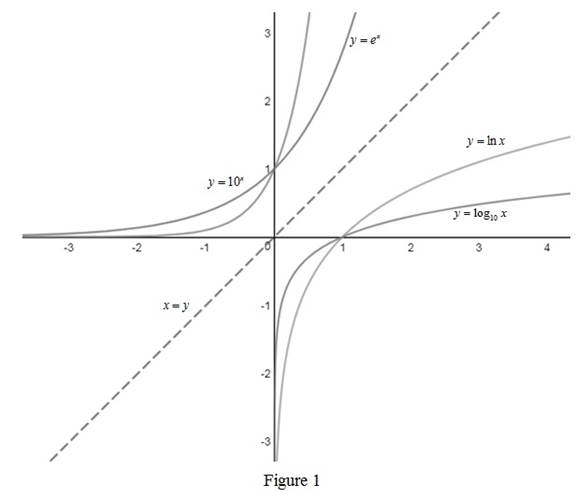# Use Formula 10 to graph the functions y = ln x , y = log 10 x , y = e x and y = 10 x on a common screen and interpret how these graphs is related.### Single Variable Calculus: Concepts...

4th Edition
James Stewart
Publisher: Cengage Learning
ISBN: 9781337687805### Single Variable Calculus: Concepts...

4th Edition
James Stewart
Publisher: Cengage Learning
ISBN: 9781337687805

#### Solutions

Chapter 1.6, Problem 44E
To determine

## To sketch: Use Formula 10 to graph the functions y=lnx,y=log10x,y=ex and y=10x on a common screen and interpret how these graphs is related.

Expert Solution

### Explanation of Solution

The graphs of the function y=lnx,y=log10x,y=ex and y=10x are drawn and shown below in Figure 1.From Figure 1, it is noted that the graph y=10x is the inverse y=log10x and the y=ex is the inverse of y=lnx as they are reflection about the line y=x .

Use Formula 10, logba=lnalnb,b1 and express the function as, log10x=lnxln10 .

Thus, the graph of log10x can be obtained by vertically compressing the graph of ln x by a factor of ln 10.

### Have a homework question?

Subscribe to bartleby learn! Ask subject matter experts 30 homework questions each month. Plus, you’ll have access to millions of step-by-step textbook answers!